# 3D Forced Convection in a Box Containing Two Cylindrical Heat Sources

3D Forced Convection in a Box Containing Two Cylindrical Heat Sources

Said Bouabdallah* Badia Ghernaout Abderrahmane Bellaouar Abdelwafi Bouras Abdehamid Ghazel

LME Laboratory of Mechanics, Department of Mechanical Engineering. University of Laghouat, Laghouat 03000, Algeria

Materials, Energy Systems Technology and Environment Laboratory, University of Ghardaia, Ghardaia 47000, Algeria

Corresponding Author Email:
s.bouabdallah@lagh-univ.dz
Page:
20-25
|
DOI:
https://doi.org/10.18280/ama_b.631-404
15 September 2019
|
Accepted:
12 June 2020
|
Published:
31 December 2020
| Citation

OPEN ACCESS

Abstract:

Forced convection is a special type of heat transfer in which fluids are forced to move, in order to increase the heat transfer. The aim of this study is to investigate in a numerical simulation of forced convection in a cubic cavity containing two cylindrical heat sources in the middle. The cavity is subjected to an inflow of air inlet from the left and an outlet flow from the right side with the same dimensions of the opening doors (0.1 H). The walls of the cavity are maintained adiabatic. The conservation equations governing the flow of forced convection are solved using the finite volume method that is used by FLUENT –ANSYS Software. Also, a comparison was performed shows good agreement between the numerical results with those available in the literature (numerical, analytical and experimental). The effect of the dimension of cavity (number of Rayleigh) and the velocity of ventilation of the air (number of Reynolds), as well as the dimension between sources cylindrical are presented and discussed. The results obtained show a strong dependence between the geometrical conditions and the structure of the dynamic and thermal field of the flow of forced convection.

Keywords:

3D flow, cooling, simulation, ventilation, cubical cavity

1. Introduction

In the last few decades, natural and forced convection in enclosures with the present of a heat sources plays an important role and has been the subject of extensive research in several engineering problems. Such as electronic cooling, air conditioning, air-cooling, nuclear reactors, food and medical industries, this practical interest explains the existence of various studies considering different configurations and boundary conditions.

Many researchers have studied natural, forced and mixed convection heat transfer in enclosures with the presence of a body and various thermal boundary conditions [1-4].

Young and Vafai  investigated in a forced convection cooling of a set of heat sources mounted on the bottom wall of a channel. The study used variations in the height and width of the source, and also the influence of the Reynolds number, the results presented show that the shape and the material of the source have considerable effects on the characteristics of the flow and heat transfer.

A numerical simulation of forced convection has been carried out by Fu and Tong . They studied the influence of an oscillating cylinder on heat transfer at a number of heat sources subjected to flow in a horizontal channel, the results show that heat transfer increases with the increasing of Reynolds number and improves remarkably for significant oscillations of the cylinder. Mohamed  investigated experimentally in order to know the characteristics of air cooling in a cooling device, four devices of 9, 16, 25 and 36 cm2 modules placed in the same region and projecting. They found that the average heat transfer coefficient increases slightly with the increase of the temperature of the device of the module, where the increase is considerably higher with increasing airflow velocities and module height. The mixed convection heat transfer characteristics within a ventilated square cavity having a heated hollow cylinder has been numerically investigated by Mamun et al. . They noted that the cylinder diameter has significant effect on both the flow and thermal fields but the solid-fluid thermal conductivity ratio has significant effect only on the thermal field.

The heat transfer by forced convection from two equal circular cylinders located at the mid-section of cavity with a square shape was examined. The obtained results showed the augmentation in heat transfer rate with increasing the distance between cylinders (Helmaoui et al. ). Laidoudi et al.  exhibited the profound influence of inlet and outlet ports on the heat transfer from the circular cylinders. Koufi et al.  also emphasized the effects of the openings in turbulent heat transfer via mixed convection inside vented enclosures. Gokulavani et al.  explored the free convection in cavity containing a heated baffle at its center positioned vertically or horizontally with different configurations of the open cavity on vertical walls, for various Rayleigh numbers, Reynolds numbers and aspect ratios. The vertical baffle was shown to be more effective for augmenting heat transfer compared to the horizontal baffle.

Furthermore, three-dimensional convection in a heated cavity some sutideds have been presented. Ouertatani et al.  investigated numerically the 3D mixed convection of air in a cubic cavity. The upper and lower walls are moving and maintained at different temperatures (heated from above and cooled down), while the other walls are adiabatic. The control parameters are Richardson numbers 0.001 ≤ Ri ≤10, Reynolds 100 ≤ Ri ≤ 1000 and Prandtl ≤ 0.71. The results indicate that a remarkable improvement in heat transfer up to 76% can be reached for the particular combination of Re = 400 and Ri = 1. Karimi et al.  Studied the simulation of forced convection in a 2D cavity, which contains two heat sources in the center the results indicate that the variation in the average Nusselt number dependent on the Reynolds number. They show that the increasing of Reynolds number increases the entropy generation, while the incorporation of nano-fluids into the base fluid greatly minimizes the entropy generation.

Experimentally and numerically, Yang et al.  investigated turbulent mixed convection in a vertical open cavity. Turbulent mixed convection in a vented cavity was undertaken in  focusing on the effect of position of inlet and outlet slots on the ventilation efficacy of air to determine the optimum configuration. The numerical results of Afolabi et al.  show that there is a significant influence of Ra number on the heat transfer thus the temperature distribution in the cavity. Numerical calculations are carried out by Hojat and Seyed  for natural convection induced by a temperature difference between a cold outer square enclosure and a hot inner cylinder with two different geometries (i.e. circular and square). The results showed that: for Ra = 105, only a uni-cellular vortex is formed in the enclosure irrespective of the position of the inner cylinder. Also, as the obtained total surfaces-averaged Nusselt numbers of the enclosure show, in all cases, at the same Rayleigh number, the rate of heat transfer from the enclosure which the circular cylinder is located inside is better. In other cases, the shape of heat source was investigated by Jelita er al. [16-18] for different geometries, elliptical, cylinder, equilateral and triangle.

In 2D ventilated enclosure containing a heat source placed in the middle, Bouabdallah et al.  show that the thermal and flow field are highly dependent to the dimension and the geometry of the heat source. They show also, that the better cooling of the heat source is obtained for the circular shape, and the flow structure, the heat transfer rate is highly dependent to the convective system and the size of the heat source.

The literature review showed the existence of a limited number of three-dimensional forced convection studies and that explains the choice of our present physical model. In the present work, we are interested to the numerical simulation of the 3D flow of forced convection in a box containing two cylindrical heat sources. The simulations were made for Rayleigh number: 2.5×107 < Ra < 9 × 107, and for a Reynolds number 2 ≤ Re ≤ 500. Section 2 presents the physical problem and mathematical formulation. Section 3 presents the results and discussion: the effect of mesh spacing, validation of the results. Next, the effect of the geometrical dimension, the velocity ventilation and the distance between the two heat sources are presented and discussed. Finally, a conclusion is given.

2. Physical Problem and Mathematical Formulation

2.1 Geometrical configuration

The physical system considered is shown schematically in Figure 1. A three-dimensional cavity of length L and height H containing heat sources in the middle (sources of different shapes and sizes). The cavity is subjected to a flow of air entering from the left (center) and coming out from the right side with the same size of the opening (0.1 H).

## 1.png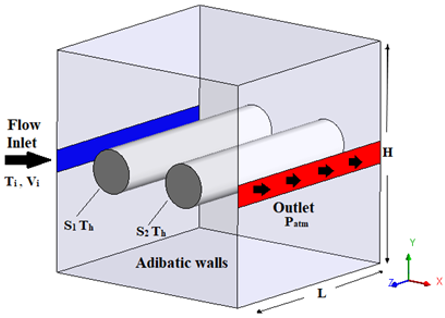Figure 1. Geometric configuration and boundary condition of the problem

2.2 General equations

The analysis of the thermal convection is done by the application of the three fundamental laws of conservation (conservation of the mass, the momentum, and the energy). The differential formulation of these equations is as follows (Helmaoui et al. ):

Mass conservation equation: According to the principle of fluid mass conservation that we follow remains constant in its movement, we can write our continuity equation as an index form:

$\frac{\partial \rho }{\partial t}+\frac{\partial }{\partial {{x}_{j}}}(\rho {{u}_{j}})=0$          (1)

Conservation equation of momentum: This equation is deduced from the second law of dynamics, which states that the variation of the momentum of a fluid particle is equal to the sum of the external forces on that particle. It is written in tensorial form as follows:

$\frac{\partial }{\partial t}(\rho {{u}_{i}})+\frac{\partial }{\partial {{x}_{j}}}(\rho {{u}_{i}}{{u}_{j}})=fi-\frac{\partial p}{\partial {{x}_{i}}}+\frac{\partial }{\partial {{x}_{j}}}\left[ u\left( \frac{\rho {{u}_{i}}}{\partial {{x}_{j}}}+\frac{\rho {{u}_{j}}}{\partial {{x}_{i}}} \right) \right]$           (2)

Energy equation: The energy equation is obtained by applying the first principle of thermodynamics for an incompressible Newtonian fluid which states that the variation of total energy (sum of the kinetic energy and the internal energy) is equal to the sum of the power of the external forces and the transfer of heat, it is written as follows:

$\frac{\partial T}{\partial t}+\frac{\partial }{\partial {{x}_{j}}}({{u}_{j}}T)=\alpha \frac{{{\partial }^{2}}T}{\partial x_{j}^{2}}+q+\mu \Phi$          (3)

To simplify the resolution of the physical problem, simplifying assumptions are introduced at the modeling level. We therefore limit ourselves to the hypotheses commonly used in studies of natural convection: Knowing that the flow is three-dimensional (3D), the physical properties of the fluid inside the cavity are assumed to be constant (except ρ which satisfies the Boussinesq approximation), and the fluid is compressible. Radiation heat transfer is also neglected and we assume that the regime is stationary and laminar.

According to x:

$\frac{\partial T}{\partial x}=0$, $u=v=w=0$; (adiabatic walls).

for $\left\{\begin{array}{l}x=0, z \\ x=H, z\end{array}\right.$ and $\left\{\begin{array}{l}0 \prec y \prec 0.45 H \\ 0.55 H \prec y \prec H\end{array}\right.$           (4)

According to y:

$u=0.1m/s$, $v=w=0$, for $x=0,z$; $0.45H\le y\le 0.55H$       (5)

According to z:

$\frac{\partial T}{\partial z}=0, u=v=w=0$; for $\left\{\begin{array}{l}z=0, x \\ y=H, x\end{array}\right.$ and $\left\{\begin{array}{l}y=H, x \\ 0 \prec z \prec H\end{array}\right.$         (6)

3. Results and Discussions

In this section we will firstly see the effect of mesh spacing on numerical computation, and then we do the compassion of our results with those available in the literature (numerical, analytical and experimental). Then, we will study the influence of several parameters on the flow field and heat transfer rates within the cavity. Such as: the dimension of the cavity and the velocity ventilation and the distance between tow cylindrical heat source.

3.1 Effect of mesh spacing

In computation the number and the form of the control volume constructed the computational domain have a great influence on the precision of the results and the calculation time [20-22]. To explore the effect of mesh spacing on numerical solutions, we tested three uniform meshes: 423, 623, 823 nodes. We hold a cubic box of dimension H = 0.6m (Ra = 8.57 × 107) and the air inlet with velocity of 0.05 m/s (Re = 350). The Table 1 shows the values of maximum velocity components u, v and w at the middle of the cavity, and the average Nusselt number.

Table 1. Parameters of the convection flow for different mesh sizes

 Mesh Umax (m/s) Vmax (m/s) Wmax (m/s) Nuavg 423 0.059 1.92 ×10-3 2.8 ×10-5 10.58 623 0.063 3.8 ×10-4 4.20 ×10-5 10.84 823 0.065 7.91×10-4 1.38×10-4 11.04

We note that the difference between the values presented is negligible for velocity U. But there is a different for the velocity components V and W. It is clear that the flow develops in the direction of the abscissa X, while the velocity component along the x-axis (U) is the only one that has put considerable values as we see in the Table 1. Whereas the comparison of the extreme values results is manifested according to this component.

In Figures 2-3, we have plotted the velocity and temperature profiles in the middle of the cavity. We note that the profiles given by the calculation domains 823 and 623 are very close and the difference is apparent at the air outlet. In the same the average Nusselt number at the heat source surface calculated in the all domains of calculations considered is almost identical. For the further results, we are interested by the medium mesh 633 because it takes less time and good results along the computations.

## 2.png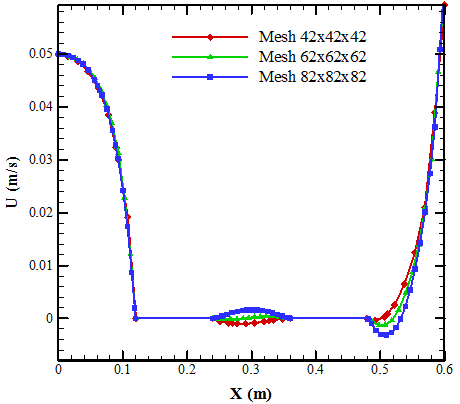Figure 2. Profiles of the horizontal velocity U component, at the middle of the cavity for different mesh sizes

## 3.pngFigure 3. Temperature profiles in the middle of the cavity for different mesh sizes

3.2 Comparison of results

Table 2. Comparison of our results with those of the literature

 Re Nuaverage on the cylinder walls Present work 3D (2019) Karimi et al. (2D), 2016  Correlation Cengel, 1998  2 0.47 1.07 1.1 20 1.95 2.53 2.56 100 5.04 5.07 5.18 200 7.73 7.06 7.2 Re McAdems, 1954  Kramers, 1946  Jain and Goel, 1976[ 26] 2 1.11 1.11 - 20 2.58 2.67 - 100 5.23 5.49 5.52 200 7.22 7.6 7.63

To give more confidence to the numerical results obtained by ANSYS software, it is imperative to compare them with the results of previous work in relation to the subject. In this work, we have made a comparison with some numerical work of Karimi et al. 2016 , and a comparison with the analytical results obtained by Cengel  and the experimental results [24-26]. On the Table 2, we find a good agreement with the results especially for a high Reynolds number (Re ≥ 100).

3.3 Effect of the cavity dimension

To see the effect of the size of the cavity on the flow field and the temperature field we vary the size of the cavity to three significant values: Ra = 2.54 x 107, Ra = 5 x107 and Ra = 8.57 x107. The Reynolds number is fixed at Re = 350.

On the Table 3, we present the average Nusselt number as a function of the Rayleigh number. We notice that the average Nusselt number increases with increasing Rayleigh. Note that the Nusselt number is the ratio of heat transfer rate (convection/conduction) at surface of cylindrical heat sources. While the rate of heat transfer increases with the increase of the box size.

In Figure 4, we presented the iso-surfaces of temperature and some fluid particles trajectory in the Box for different Rayleigh numbers. We notice that each time Ra increases, the blue zone widens, that is to say, cooled better and we see that the dynamic structure of the flow strongly depends on the geometric dimension of the cavity.

Table 3. Nusselt number for different Rayleigh numbers

 Ra 2.54 ×107 5×107 8.57×107 Nuavrage 10.33 10.83 10.84

## 4.png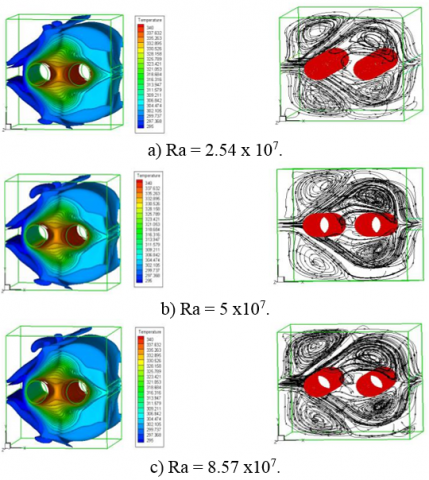Figure 4. Iso- surfaces of temperature (left) and some fluid particles trajectory in the 3D cavity (right), for different Rayleigh numbers

3.4 Effect of Reynolds number

In this section, we study the effect of the fan speed (Reynolds number) on box cooling. We holding the Rayleigh number fixed to Ra = 8.57×107 and vary the Reynolds number: Re = 2, 20, 100, 250, 350 and 500.

On the Table 4, we give the Nusselt number as a function of Reynolds number. We note that the number of Nusselt increases with the increase in the Re. So, the ventilation of the box is preferred for the increase of Re number.

Table 4. Nusselt number for different Reynolds numbers

 Re 2 20 100 250 350 500 Nu 0.42 1.95 5.04 8.52 10.84 13.72

In Figure 5, we have the iso-surfaces of temperature and some fluid particle trajectories for different Reynolds number. We note a strong dependence of the dynamic and thermal structure of the flow as a function of the ventilation rate (Reynolds number).

In Figure 6-a, we have illustrated velocity component profiles U at the center of the cavity, for different Reynolds numbers. It is found that the initial speed begins to decrease until it is broken at the sources because of the conditions of adhesion. Then the air comes out with a great speed, and that speed increases with the increase of the Re. This can be explained by the fact that air gains a quantity of heat when passing through hot springs. Similarly, we find that the temperature of the outgoing air decreases with the increase of the number of Reynolds (Figure 6-b).

## 5.png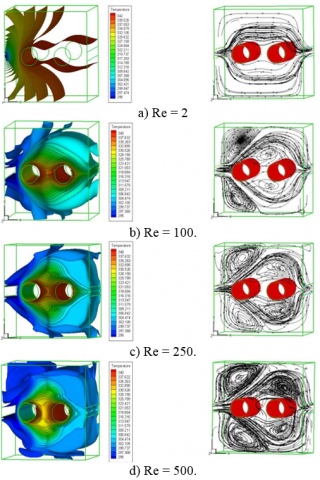Figure 5. Iso- surfaces of temperature (left) and some fluid particles trajectory in the 3D cavity (right) for different Reynolds number

## 6a.png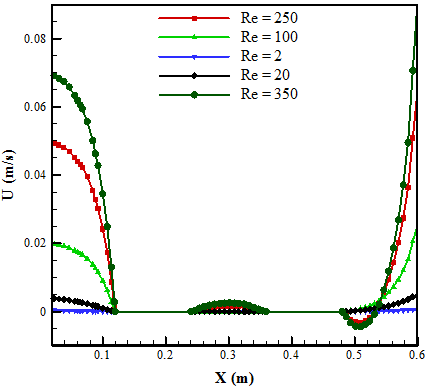(a)

## 6b.png(b)

Figure 6. U-velocity component profiles (a) and temperature profiles (b) at the middle of the cavity for different Reynolds numbers

3.5 Effect of distance between sources

To show the effect of heat transfer distribution between the cylindriacal heat source we make a study for three differents distiance between them. In Table 5, we have presented the Nusselt number as a function of the distance between the cylindrical sources denoted S (Ra = 8.57×107, Re = 350). We notice that S increases the mean Nusselt number also increased. Also, the heat transfer rate increases with the increase in the distance between them. This can be explained by the good distribution of the heat discharged by the sources when S increases.

Table 5. Nusselt number vs distance between the sources

 Distance S 0.1 0.2 0.3 Nu 10.32 10.84 11.89
4. Conclusion

Forced convection in a cubical cavity containing two cylindrical heat sources in the middle was numerically carried out. The problem is studied for different dimensions of the cavity, and for different ventilation velocity (Re). The results obtained in this work are in good agreement with previous work; and the main obtaining results can be given as follows:

• The dynamic and thermal structure of the flow depends strongly to the geometric dimension of the cavity.

• A strong dependence of the flow structure as a function of the ventilation rate.

• The heat convection rate increases with the increase of the ventilation flow.

• These results can be used in further investigation to improve the industrial system, in particular, the cooling of electronic components and devices.

• In the future, we propose to develop an experimental model and make it available for other advances.

Acknowledgment

The authors gratefully acknowledge the Directorate General of Scientific Research and Technological Development (DGSRTD) – to support this work, and fund the LME laboratory for carrying out research.

Nomenclature
 Cp Specific heat, J. kg-1. K-1 k Thermal conductivity, W.m-1. K-1 Nu Nusselt number H Height of cavity, m L Length of cavity, m Ra Rayleigh number Re Reynolds number u, v, w Velocity components, m. s-1 S Distance between the heat sources T Temperature, K x, y, z Cartesian coordinates, m Greek symbols $\alpha$ Thermal diffusivity, m2. s-1 $\beta$ Thermal expansion coefficient, K-1 ρ Density of fluid, kg. m-3 µ Dynamic viscosity, kg. m-1.s-1 Subscripts avg average c cold h hot
References

 Young, T.J., Vafai, K. (1998). Convective cooling heated obstacle in a channel. International Journal of Heat and Mass Transfer, 41(20): 3131-3148. https://doi.org/10.1016/S0017-9310(97)00323-2

 Fu, W.S., Tong, B.H. (2004). Numerical investigation of heat transfer characteristics of the heated blocks in the channel with transversally oscillating cylinder. International Journal of Heat Transfer, 47(2): 341-351. https://doi.org/10.1016/S0017-9310(03)00303-X

 Mohamed, M.M. (2006). Air cooling characteristics of a uniform square modules array for electronic device heat sink. Applied Thermal Engineering, 26(5): 486-493. https://doi.org/10.1016/j.applthermaleng.2005.07.013

 Hsu, T.H., How, S.P. (1999). Mixed convection in an enclosure with a heat-conducting body. Acta Mechanica, 133(1): 87-104. https://dx.doi.org/10.1007/BF01179012

 Mamun, M.A.H., Rahman, M.M., Billah, M.M., Saidur, R. (2010). A numerical study on the effect of a heated hollow cylinder on mixed convection in a ventilated cavity. International Communications in Heat and Mass Transfer, 37(9): 1326-1334. https://dx.doi.org/10.1016/j.icheatmasstransfer.2010.07.019

 Helmaoui, M., Laidoudi, H., Belbachir, A., Ayad, A., Ghaniam, A. (2020). Forced convection heat transfer from a pair of circular cylinders confined in ventilated enclosure. Diffusion Foundations, 26: 104-111 http://dx.doi.org/10.4028/www.scientific.net/DF.26.104

 Laidoudi, H., Helmaoui, M., Belbachir, A., Ayad, A., Ghaniam, A., (2020). Effects of inlet and outlet ports of ventilated square cavity on flow and heat transfer. Diffusion Foundations, 26: 78-85. http://dx.doi.org/10.4028/www.scientific.net/DF.26.78

 Koufi, L., Younsi, Z., Cherif, Y., Naji, H. (2017). Numerical investigation of turbulent mixed convection in an open cavity: Effect of inlet and outlet openings. International Journal of Thermal Sciences, 116: 103-117. http://dx.doi.org/10.1016/j.ijthermalsci.2017.02.007

 Gokulavani, P., Muthtamilselvan, M., Qasem, M.A., Doh, D.H. (2020). Effects of orientation of the centrally placed heated baffle in an alternative configured ventilation cavity. European Physical Journal Plus, 135(23). http://dx.doi.org/10.1140/epjp/s13360-019-00070-7

 Ouertatani, N., Ben Cheikh, N., Ben Bey, B., Lili, T., Campo, A. (2009). Mixed convection in a double lid-driven cubic cavity. International Journal of Thermal Sciences, 48(7): 1265-1272. http://dx.doi.org/10.1016/j.ijthermalsci.2008.11.020

 Karimi, F., Xu, H.T., Wang, Z., Yang, M. (2016). Numerical simulation of transient forced convection in a square enclosure containing two heated circular cylinders. International Journal of Numerical Methods for Heat & Fluid Flow, 26(1): 307-327. http://dx.doi.org/10.1108/HFF-09-2014-0281

 Yang, G., Huang, Y., Wu, J., Zhang, L., Chen, G., Lv, R., Cai, A. (2017). Experimental study and numerical models’ assessment of turbulent mixed convection heat transfer in a vertical open cavity. Building and Environment, 115: 91-103. http://dx.doi.org/10.1016/j.buildenv.2017.01.016

 Koufi, L., Younsi, Z., Cherif, Y., Naji, H. (2017). Numerical investigation of turbulent mixed convection in an open cavity: Effect of inlet and outlet openings. International Journal of Thermal Sciences, 116: 103-117. http://dx.doi.org/10.1016/j.ijthermalsci.2017.02.007

 Afolabi, S.I., Ojo, A.O., Oluleye, M.A., Ojo, A.A. (2019). Convective heat transfer in a square cavity with a heat-generating body of different aspect ratios. International Journal of Engineering Research & Technology, 8(6): 555-562. http://dx.doi.org/10.17577/IJERTV8IS060409

 Hojat, K., Seyed, A.M. (2012). Comparison of natural convection a round a circular cylinder with a square cylinder inside a square enclosure. Journal of Mechanical Engineering and Automation, 2(6): 176-183. http://dx.doi.org/10.5923/j.jmea.20120206.08

 Jelita, M., Mudia, H., Afriani, S. (2017). Analysis of fluid dynamics and heat transfer inside an elliptical cylinder in a square enclosure. Advances in Theoretical and Applied Mechanics, 10(1): 11-20. https://doi.org/10.12988/atam.2017.752

 Altaee, A.H., Hassan Ali, F., Mahdi, Q.A. (2017). Natural convection inside square enclosure containing equilateral triangle with different orientations. Journal of Babylon University Engineering Sciences, 4(25): 1194-1205.

 Gibanov, N., Sheremet, M., (2017). Unsteady natural convection in a cubical cavity with a triangular heat source. International Journal of Numerical Methods for Heat & Fluid Flow, 27(8): 1795-1813. http://dx.doi.org/10.1108/HFF-06-2016-0234

 Bouabdallah, S., Chati, D., Ghernaout, B., Atia, A., Laouirate, A. (2016). Turbulent mixed convection in enclosure containing a circular/square heat source. International Journal of Heat & Technology, 34(3): 446-454. http://dx.doi.org/10.18280/ijht.340314

 Atia, A., Ghernaout, B., Bouabdallah, S. (2018). Transition from steady to oscillatory flow natural convection of low-Pr fluids in 3D Bridgman configuration for crystal growth. Journal of Applied Fluid Mechanics, 11(4): 1021-1031. https://doi.org/10.29252/jafm.11.04.27603

 Ghernaout, B., Attia, M.H.A., Bouabdallah, S., Driss, Z., Benali, M.L. (2020). Heat and fluid flow in an agricultural greenhouse. International Journal of Heat & Technology, 38(1): 92-98. http://dx.doi.org/10.18280/ijht.380110

 Ghernaout, B., Bouabdallah, S., Attia, M.H.A, Arıcı, M., Driss, Z. (2020). Parametric study of the airflow structure in a solar chimney. International Journal of Heat & Technology, 38(2): 285-292. http://dx.doi.org/10.18280/ijht.380202

 Cengel, Y.A. (1998). Heat Transfer: A Practical Approach, WCB McGraw Hill, Boston, MA.

 McAdams, W.H. (1954). Heat Transmission, McGraw Hill, New York, NY.

 Kramers, H.A. (1946). Heat transfer from spheres to flowing media. Physics, 12(12): 61-80. http://dx.doi.org/10.1016/S0031-8914(46)80024-7

 Jain, P.C., Goel, B.S. (1976). A numerical study of unsteady laminar forced convection from a circular cylinder. ASME Journal of Heat Transfer, 98(2): 303-307. http://dx.doi.org/10.1115/1.3450537